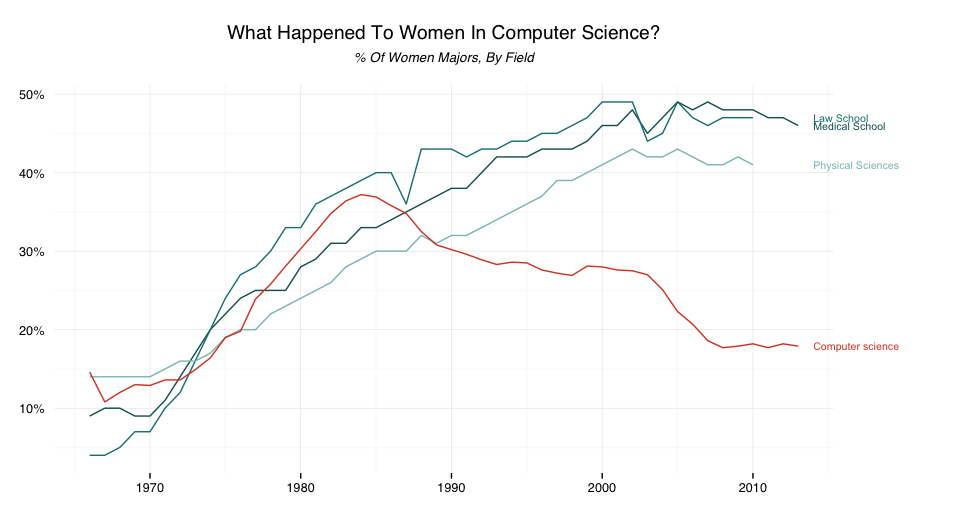This is an attempt to continue the work that @hrbrmstr did at Coloring (and Drawing) Outside the Lines in ggplot.

It all started with a tweet asking for a way to replicate this graphic in ggplot.This is the plot that @hrbrmstr created (in ggplot) at the end of his post.I took his code, and tweaked it to get the ggplot version to be as similar as possible to the original plot. Here is what I achieved:Code (some of it is taken from the @hrbrmstr’s blog post):

(Code is also available at github.com/sainathadapa)

``````library(ggplot2)
library(dplyr)
library(tidyr)
library(stringr)
library(scales)
library(gridExtra)
library(grid)
library(Cairo)
library(gtable)

# use the NPR story data file ---------------------------------------------
# and be kind to NPR's bandwidth budget
url <- "http://apps.npr.org/dailygraphics/graphics/women-cs/data.csv"
fil <- "gender.csv"

# take a look at the CSV structure ----------------------------------------

glimpse(gender)

tail(gender)

# via http://apps.npr.org/dailygraphics/graphics/women-cs/js/graphic.js
# there are 'tk' values in the data set that should be ignored so
# replace them with NA by ensuring all columns are numeric.
# also the color values came from that javascript file, too.

gender <- mutate_each(gender, funs(as.numeric))

# make better column labels for display ----------------------------------

colnames(gender) <- str_replace(colnames(gender), "\\.", " ")

gender_long <- mutate(gather(gender, area, value, -date),
area=factor(area, levels=colnames(gender)[2:5],
ordered=TRUE))

gender_colors <- c('#11605E', '#17807E', '#8BC0BF','#D8472B')
names(gender_colors) <- colnames(gender)[2:5]

# ggplot ------------------------------------------------------------------

gg <- ggplot(gender_long)

# Using LOESS smoothing for the lines
gg <- gg + geom_smooth(aes(x = date, y = value, group = area, color = area),
se = F, method = "loess", span = 0.15, size = 0.85) +
geom_hline(yintercept = 0, size = 0.2) +
scale_color_manual(name = "", values = gender_colors) +
scale_y_continuous(label = percent,
breaks = seq(from = 0,to = 0.5,by = 0.05)) +
scale_x_continuous(breaks = seq(from = 1970, to = 2010, by = 5)) +
coord_cartesian(xlim = c(1966, 2013), ylim = c(0, 0.55)) +
labs(x = NULL, y = NULL, title = NULL)

# Theme settings
gg <- gg + theme_bw(base_family = "Helvetica", base_size = 10)
gg <- gg + theme(axis.ticks.y          = element_blank(),
panel.border          = element_blank(),
legend.key            = element_blank(),
legend.justification  = 'left',
legend.position       = c(-0.075,1.01),
legend.direction      = 'horizontal'),
legend.key.height     = unit(0.1, 'cm'),
legend.key.width      = unit(0.1, 'cm'),
panel.grid            = element_line(linetype = 'dotted',
size     = 1,
color    = 'black'))
gg <- gg + guides(colour = guide_legend(override.aes = list(size = 5)))

# Needed to use viewports to align and size the text properly
vplayout <- function(x, y) viewport(layout.pos.row = x, layout.pos.col = y)

gg1 <- textGrob(expression(bold("What Happened To Women In Computer Science?")),
vp    = vplayout(1,1),
x     = unit(0.02, "npc"),
y     = unit(0.5, "npc"),
hjust = 0,
vjust = 0,
gp    = gpar(fontsize = 12, face = "bold", col = "black"))

gg2 <- textGrob("% Of Women Majors, By Field",
vp    = vplayout(2,1),
hjust = 0,
vjust = -0.5,
x     = unit(0.02, "npc"),
y     = unit(1, "npc"),
just  = "left",
gp    = gpar(fontsize = 10,col = "black"))
gg3 <- gg

gg4 <- textGrob("Source: National Science Foundation, American Bar Association, American Association of Medical Colleges",
x     = unit(0.02, "npc"),
y     = unit(0, "npc"),
hjust = 0,
vjust = -0.5,
vp    = vplayout(15,1),
gp    = gpar(fontsize = 8, col = "black"))

gg5 <- textGrob("Credit: Quoctrung Bui/NPR",
x     = unit(0.02, "npc"),
y     = unit(0, "npc"),
hjust = 0,
vjust = -1,
vp    = vplayout(16,1),
gp    = gpar(fontsize = 8, col = "black"))

layout_matrix <- matrix(c(1,1, 2,2, rep(3, times = 20), 4, 5),ncol = 1)

# arrangeGrob (as the name says) to arrange the all the different elements
ggf <- arrangeGrob(gg1, gg2, gg3, gg4, gg5, layout_matrix = layout_matrix)

grid.draw(ggf)
``````